Courses

# Power Series And Functions Mathematics Notes | EduRev

## Mathematics: Power Series And Functions Mathematics Notes | EduRev

The document Power Series And Functions Mathematics Notes | EduRev is a part of the Mathematics Course Algebra for IIT JAM Mathematics.
All you need of Mathematics at this link: Mathematics

We opened the last section by saying that we were going to start thinking about applications of series and then promptly spent the section talking about convergence again. It’s now time to actually start with the applications of series.
With this section we will start talking about how to represent functions with power series. The natural question of why we might want to do this will be answered in a couple of sections once we actually learn how to do this.
Let’s start off with one that we already know how to do, although when we first ran across this series we didn’t think of it as a power series nor did we acknowledge that it represented a function.
Recall that the geometric series is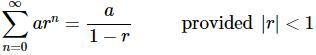(1)
Don’t forget as well that if |r|≥1 the series diverges.
Now, if we take a=1 and r=x this becomes,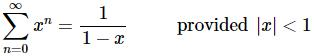Turning this around we can see that we can represent the function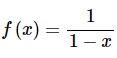(2)
with the power series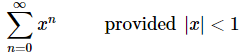This provision is important. We can clearly plug any number other than x=1 into the function, however, we will only get a convergent power series if |x|<1. This means the equality in (1) will only hold if |x|<1. For any other value of x the equality won’t hold. Note as well that we can also use this to acknowledge that the radius of convergence of this power series is R=1 and the interval of convergence is |x|<1.
This idea of convergence is important here. We will be representing many functions as power series and it will be important to recognize that the representations will often only be valid for a range of x’s and that there may be values of x that we can plug into the function that we can’t plug into the power series representation.
In this section we are going to concentrate on representing functions with power series where the functions can be related back to (2).
In this way we will hopefully become familiar with some of the kinds of manipulations that we will sometimes need to do when working with power series.
So, let’s jump into a couple of examples.

Example 1: Find a power series representation for the following function and determine its interval of convergence.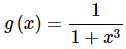Solution. What we need to do here is to relate this function back to (2). This is actually easier than it might look. Recall that the x in (2) is simply a variable and can represent anything. So, a quick rewrite of g(x) gives,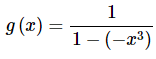and so the −x3 in g(x) holds the same place as the x in (2). Therefore, all we need to do is replace the x in (3) and we’ve got a power series representation for g(x).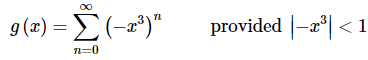Notice that we replaced both the x in the power series and in the interval of convergence.
All we need to do now is a little simplification.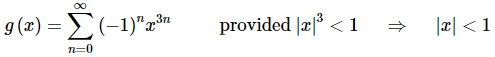So, in this case the interval of convergence is the same as the original power series. This usually won’t happen. More often than not the new interval of convergence will be different from the original interval of convergence.

Example 2: Find a power series representation for the following function and determine its interval of convergence.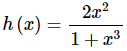Solution. This function is similar to the previous function. The difference is the numerator and at first glance that looks to be an important difference. Since (2) doesn’t have an x in the numerator it appears that we can’t relate this function back to that.
However, now that we’ve worked the first example this one is actually very simple since we can use the result of the answer from that example. To see how to do this let’s first rewrite the function a little.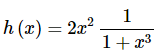Now, from the first example we’ve already got a power series for the second term so let’s use that to write the function as,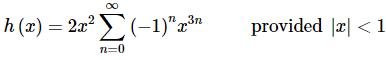Notice that the presence of x’s outside of the series will NOT affect its convergence and so the interval of convergence remains the same.
The last step is to bring the coefficient into the series and we’ll be done. When we do this make sure and combine the x’s as well. We typically only want a single x in a power series.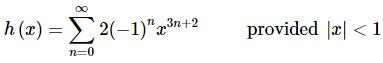As we saw in the previous example we can often use previous results to help us out. This is an important idea to remember as it can often greatly simplify our work.

Example 3: Find a power series representation for the following function and determine its interval of convergence.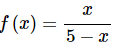Solution. So, again, we’ve got an x in the numerator. So, as with the last example let’s factor that out and see what we’ve got left.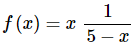If we had a power series representation for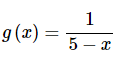we could get a power series representation for f(x).
So, let’s find one. We’ll first notice that in order to use (2) we’ll need the number in the denominator to be a one. That’s easy enough to get.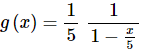Now all we need to do to get a power series representation is to replace the x in (3) with x/5. Doing this gives,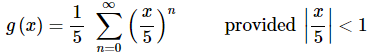Now let’s do a little simplification on the series.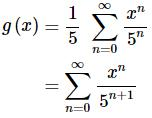The interval of convergence for this series is,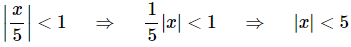Okay, this was the work for the power series representation for g(x) let’s now find a power series representation for the original function. All we need to do for this is to multiply the power series representation for g(x) by x and we’ll have it.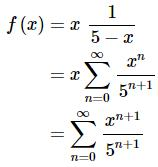The interval of convergence doesn’t change and so it will be |x|<5.
So, hopefully we now have an idea on how to find the power series representation for some functions. Admittedly all of the functions could be related back to (2) but it’s a start.
We now need to look at some further manipulation of power series that we will need to do on occasion. We need to discuss differentiation and integration of power series.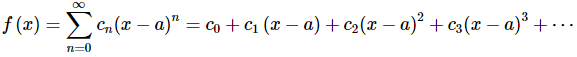Now, we know that if we differentiate a finite sum of terms all we need to do is differentiate each of the terms and then add them back up. With infinite sums there are some subtleties involved that we need to be careful with but are somewhat beyond the scope of this course.
Nicely enough for us however, it is known that if the power series representation of f(x) has a radius of convergence of R>0 then the term by term differentiation of the power series will also have a radius of convergence of R and (more importantly) will in fact be the power series representation of f′(x) provided we stay within the radius of convergence.
Again, we should make the point that if we aren’t dealing with a power series then we may or may not be able to differentiate each term of the series to get the derivative of the series.
So, what all this means for us is that,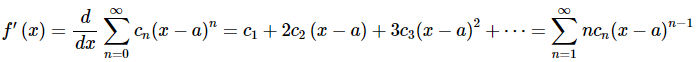Note the initial value of this series. It has been changed from n=0 to n=1. This is an acknowledgement of the fact that the derivative of the first term is zero and hence isn’t in the derivative. Notice however, that since the n=0 term of the above series is also zero, we could start the series at n=0 if it was required for a particular problem. In general, however, this won’t be done in this class.
We can now find formulas for higher order derivatives as well now.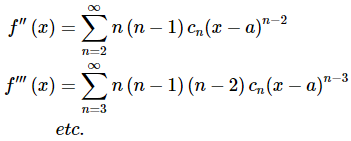Once again, notice that the initial value of n changes with each differentiation in order to acknowledge that a term from the original series differentiated to zero.
Let’s now briefly talk about integration. Just as with the differentiation, when we’ve got an infinite series we need to be careful about just integration term by term. Much like with derivatives it turns out that as long as we’re working with power series we can just integrate the terms of the series to get the integral of the series itself. In other words,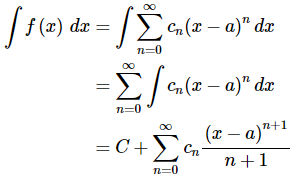Notice that we pick up a constant of integration, C, that is outside the series here.
Let’s summarize the differentiation and integration ideas before moving on to an example or two.
Fact
If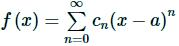has a radius of convergence of R>0 then,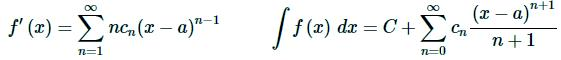and both of these also have a radius of convergence of R.
Now, let’s see how we can use these facts to generate some more power series representations of functions.

Example 4: Find a power series representation for the following function and determine its interval of convergence.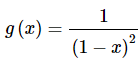Solution. To do this problem let’s notice that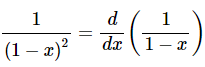Then since we’ve got a power series representation for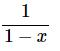all that we’ll need to do is differentiate that power series to get a power series representation for g(x).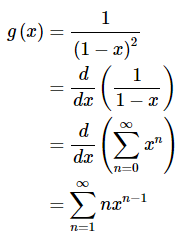Then since the original power series had a radius of convergence of R=1 the derivative, and hence g(x), will also have a radius of convergence of R=1.

Example 5: Find a power series representation for the following function and determine its interval of convergence.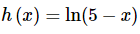Solution. In this case we need to notice that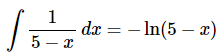and then recall that we have a power series representation for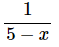Remember we found a representation for this in Example 3. So,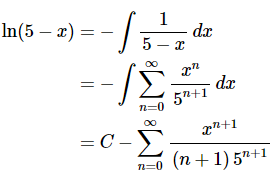We can find the constant of integration, C, by plugging in a value of x. A good choice is x=0 since that will make the series easy to evaluate.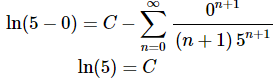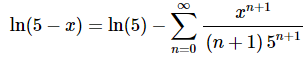Note that it is okay to have the constant sitting outside of the series like this. In fact, there is no way to bring it into the series so don’t get excited about it.

The document Power Series And Functions Mathematics Notes | EduRev is a part of the Mathematics Course Algebra for IIT JAM Mathematics.
All you need of Mathematics at this link: MathematicsUse Code STAYHOME200 and get INR 200 additional OFF Use Coupon Code
All Tests, Videos & Notes of Mathematics: Mathematics## Algebra for IIT JAM Mathematics

70 videos|58 docs

Track your progress, build streaks, highlight & save important lessons and more!

,

,

,

,

,

,

,

,

,

,

,

,

,

,

,

,

,

,

,

,

,

;